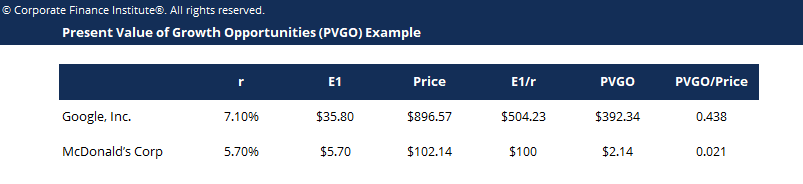# Present Value of Growth Opportunities Template

## Present Value of Growth Opportunities Template

This present value of growth opportunities template shows you how to calculate the PVGO given the market price of stock, required rate of return and expected earnings.

This is what the PVGO template looks like:### PVGO Template

Present Value of Growth Opportunities (PVGO) is a concept that gives analysts a different approach to equity valuation. Considering that valuation in stock markets is a combination of fundamentals and expectations, we can break down the value of a stock to the sum of (i) its value assuming no earnings reinvested and (ii) the present value of growth opportunities.

### PVGO formula

We can write it down in the following form:

Value of stock = value no growth + present value of GO

Or we can restate as:

PVGO = Value of stock – value no growth  and  PVGO = Value of stock – (earnings / cost of equity)

This approach uses the assumption that companies should distribute earnings among shareholders if no better use for it can be found, such as investing in positive Net Present Value (NPV) projects.

We can call the scenario in which a company has no positive NPV projects as a no-growth scenario, and formulate the following:

Value no growth = div / (required return on equity – growth)

where dividends represent 100% of earnings, making div = earnings for this assumption, and growth = 0.

Therefore, we can rewrite the formula as:

Value no growth = earnings / required return on equity

### More Free Templates

For more resources, check out our business templates library to download numerous free Excel modeling, PowerPoint presentation and Word document templates.

• Excel Modeling Templates
• PowerPoint Presentation Templates
• Transaction Document Templates

### Financial Analyst Certification

Become a certified Financial Modeling and Valuation Analyst (FMVA)® by completing CFI’s online financial modeling classes and training program!